SSC CGL Previous Year Questions: Trigonometry - 1

# SSC CGL Previous Year Questions: Trigonometry - 1 - SSC CGL

Test Description

## 20 Questions MCQ Test SSC CGL (Tier - 1) - Previous Year Papers (Topic Wise) - SSC CGL Previous Year Questions: Trigonometry - 1

SSC CGL Previous Year Questions: Trigonometry - 1 for SSC CGL 2023 is part of SSC CGL (Tier - 1) - Previous Year Papers (Topic Wise) preparation. The SSC CGL Previous Year Questions: Trigonometry - 1 questions and answers have been prepared according to the SSC CGL exam syllabus.The SSC CGL Previous Year Questions: Trigonometry - 1 MCQs are made for SSC CGL 2023 Exam. Find important definitions, questions, notes, meanings, examples, exercises, MCQs and online tests for SSC CGL Previous Year Questions: Trigonometry - 1 below.
Solutions of SSC CGL Previous Year Questions: Trigonometry - 1 questions in English are available as part of our SSC CGL (Tier - 1) - Previous Year Papers (Topic Wise) for SSC CGL & SSC CGL Previous Year Questions: Trigonometry - 1 solutions in Hindi for SSC CGL (Tier - 1) - Previous Year Papers (Topic Wise) course. Download more important topics, notes, lectures and mock test series for SSC CGL Exam by signing up for free. Attempt SSC CGL Previous Year Questions: Trigonometry - 1 | 20 questions in 20 minutes | Mock test for SSC CGL preparation | Free important questions MCQ to study SSC CGL (Tier - 1) - Previous Year Papers (Topic Wise) for SSC CGL Exam | Download free PDF with solutions
 1 Crore+ students have signed up on EduRev. Have you?
SSC CGL Previous Year Questions: Trigonometry - 1 - Question 1

### If cosec2θ = sec(3θ – 15°), then θ is equal to:    (SSC CGL-2018)

Detailed Solution for SSC CGL Previous Year Questions: Trigonometry - 1 - Question 1

sec (3θ - 15°) = cosec 2θ
sin 2θ = cos (3θ - 15°)
cos (90° - 2θ) = cos (3θ -15°)
90° - 2θ = 3θ - 15°
5θ = 90° + 15°
θ = 21°

SSC CGL Previous Year Questions: Trigonometry - 1 - Question 2

### The value of sin2 32° + sin258° – sin30° + sec260° is equal to:    (SSC CGL-2018)

Detailed Solution for SSC CGL Previous Year Questions: Trigonometry - 1 - Question 2

sin2 32° + sin2 58° - sin 30° + sec2 60°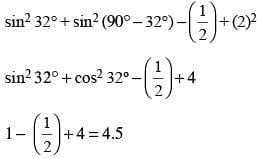SSC CGL Previous Year Questions: Trigonometry - 1 - Question 3

###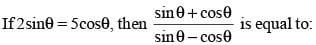(SSC CGL-2018)

Detailed Solution for SSC CGL Previous Year Questions: Trigonometry - 1 - Question 3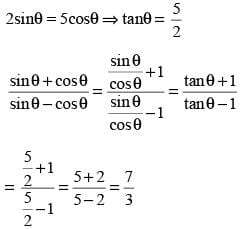SSC CGL Previous Year Questions: Trigonometry - 1 - Question 4

The value of sin238° + sin252° + sin230° – tan245° is equal to:      (SSC CGL-2018)

Detailed Solution for SSC CGL Previous Year Questions: Trigonometry - 1 - Question 4

sin238° + sin252° + sin230° – tan245°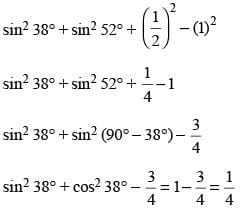SSC CGL Previous Year Questions: Trigonometry - 1 - Question 5

If sec 4θ = cosec(θ + 20°), then θ is equal to:    (SSC CGL-2018)

Detailed Solution for SSC CGL Previous Year Questions: Trigonometry - 1 - Question 5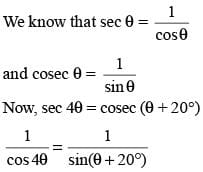sin (θ + 20°) = cos 4θ
sin (θ + 20°) = sin (90° – 4θ)
θ + 20 = 9° – 4θ
θ + 20° = 90° – 4θ

SSC CGL Previous Year Questions: Trigonometry - 1 - Question 6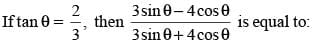(SSC CGL-2018)

Detailed Solution for SSC CGL Previous Year Questions: Trigonometry - 1 - Question 6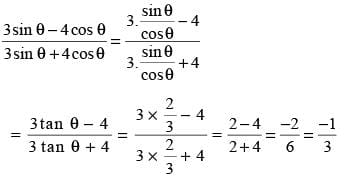SSC CGL Previous Year Questions: Trigonometry - 1 - Question 7

It is given that,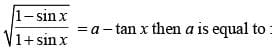(SSC CGL-2018)

Detailed Solution for SSC CGL Previous Year Questions: Trigonometry - 1 - Question 7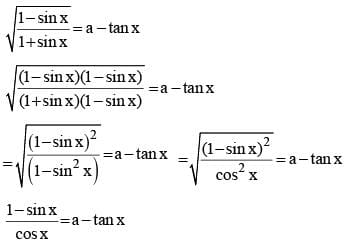sec x – tan x = a – tan x
∴ a = sec x.

SSC CGL Previous Year Questions: Trigonometry - 1 - Question 8

For α and β both being acute angles, it is given that sin (α + β) = 1, cos (α – β) = 1/2. The values of a and b are :       (SSC CHSL-2018)

Detailed Solution for SSC CGL Previous Year Questions: Trigonometry - 1 - Question 8

sin (α + β) = 1 = sin 90°
∴ α + β = 90° ...(i)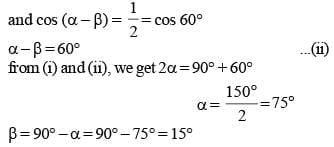SSC CGL Previous Year Questions: Trigonometry - 1 - Question 9

If θ = 9º, then what is the value of cot θ cot 2 θ cot 3 θ cot 4 θ cot 5 θ cot 6 θ  cot 7 θ cot 8 θ cot 9 θ?    (SSC CHSL-2018)

Detailed Solution for SSC CGL Previous Year Questions: Trigonometry - 1 - Question 9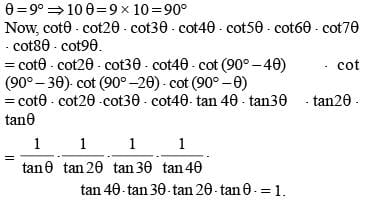SSC CGL Previous Year Questions: Trigonometry - 1 - Question 10

From the top of a 12 m high building, the angle of elevation of the top of a tower is 60° and the angle of depression of the foot of the tower is q, such that tan q = 3/4. What is the height of the tower ( 3 = 1.73)?    (SSC Sub. Ins. 2018)

Detailed Solution for SSC CGL Previous Year Questions: Trigonometry - 1 - Question 10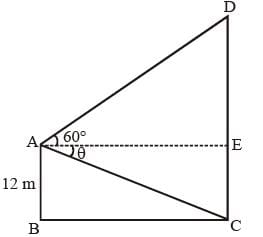Let AB is the height of the building and CD is the height of the tower.
Angle of elevation ∠DAE = 60 ° and angle of depression ∠CAE = θ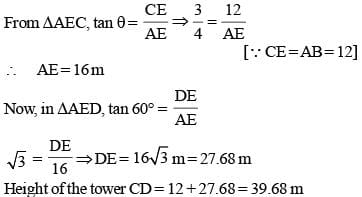SSC CGL Previous Year Questions: Trigonometry - 1 - Question 11

If tan 3x = cot (30° + 2x), then what is the value of x?     (SSC Sub. Ins. 2018)

Detailed Solution for SSC CGL Previous Year Questions: Trigonometry - 1 - Question 11

tan 3x = cot (30° + 2x)
cot(90° – 3x) = cot(30° + 2x)
90° – 3x = 30° + 2x
60° = 2x + 3x ⇒ 5x = 60°
x = 12°

SSC CGL Previous Year Questions: Trigonometry - 1 - Question 12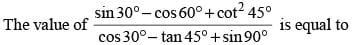(SSC Sub. Ins. 2018)

Detailed Solution for SSC CGL Previous Year Questions: Trigonometry - 1 - Question 12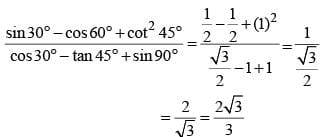SSC CGL Previous Year Questions: Trigonometry - 1 - Question 13

A ladder leaning against a wall makes an angle q with the horizontal ground such that sin θ = 12/13. If the foot of the ladder is 7.5 m from the wall, then what is the height of the point where the top of the ladder touches the wait?    (SSC Sub. Ins. 2018)

Detailed Solution for SSC CGL Previous Year Questions: Trigonometry - 1 - Question 13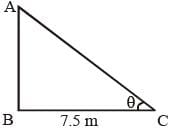Let AC is a ladder and AB is the height of the wall.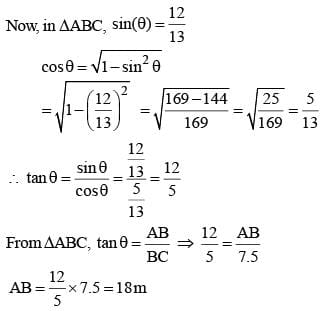SSC CGL Previous Year Questions: Trigonometry - 1 - Question 14

If θ + φ = 2/3 π and cos θ = √3/2, then what is the value of sin φ?    (SSC  Sub. Ins. 2017)

Detailed Solution for SSC CGL Previous Year Questions: Trigonometry - 1 - Question 14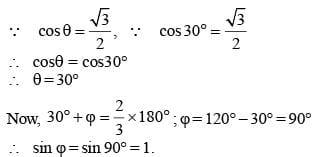SSC CGL Previous Year Questions: Trigonometry - 1 - Question 15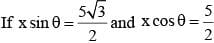then what is the value of x?    (SSC  Sub. Ins. 2017)

Detailed Solution for SSC CGL Previous Year Questions: Trigonometry - 1 - Question 15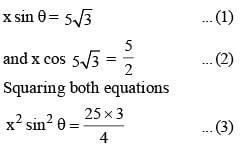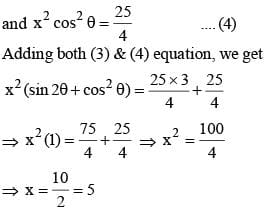SSC CGL Previous Year Questions: Trigonometry - 1 - Question 16

If sin (θ + 23°) = cos 58°, then what is the value of cos 5 θ?    (SSC  Sub. Ins. 2017)

Detailed Solution for SSC CGL Previous Year Questions: Trigonometry - 1 - Question 16

sin(θ + 23°) = cos 58°
sin (θ + 23°) = sin (90° – 58°)
sin (θ + 23°) = sin 32°
θ + 23° = 32°
θ = 32° – 23° = 9°
cos 58 = cos (5 × 9°) = cos 45° = 1/2

SSC CGL Previous Year Questions: Trigonometry - 1 - Question 17

If sec θ + cosec θ = √2 sec (90 – θ) then what is the value of cot θ?    (SSC  Sub. Ins. 2017)

Detailed Solution for SSC CGL Previous Year Questions: Trigonometry - 1 - Question 17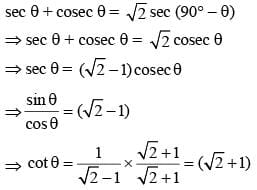SSC CGL Previous Year Questions: Trigonometry - 1 - Question 18

What is the simplified value of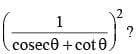(SSC  Sub. Ins. 2017)

Detailed Solution for SSC CGL Previous Year Questions: Trigonometry - 1 - Question 18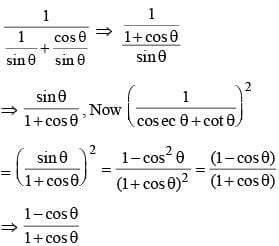SSC CGL Previous Year Questions: Trigonometry - 1 - Question 19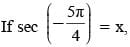then value of x is (SSC CGL 2017)

Detailed Solution for SSC CGL Previous Year Questions: Trigonometry - 1 - Question 19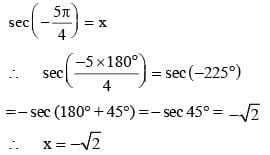SSC CGL Previous Year Questions: Trigonometry - 1 - Question 20

If 2sec A – (1 + sinA)/cos A = x, then the value of x is    (SSC  CGL 2017)

Detailed Solution for SSC CGL Previous Year Questions: Trigonometry - 1 - Question 20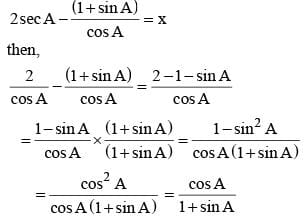## SSC CGL (Tier - 1) - Previous Year Papers (Topic Wise)

250 tests
Information about SSC CGL Previous Year Questions: Trigonometry - 1 Page
In this test you can find the Exam questions for SSC CGL Previous Year Questions: Trigonometry - 1 solved & explained in the simplest way possible. Besides giving Questions and answers for SSC CGL Previous Year Questions: Trigonometry - 1, EduRev gives you an ample number of Online tests for practice

250 tests